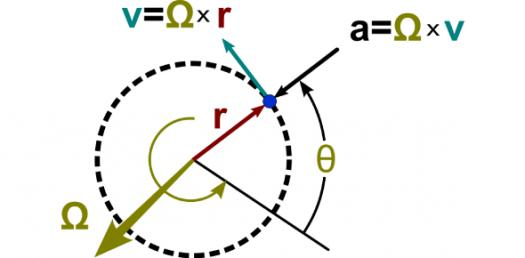# AP Physics: Circular Motion Questions! Trivia Quiz

Approved & Edited by ProProfs Editorial Team
The editorial team at ProProfs Quizzes consists of a select group of subject experts, trivia writers, and quiz masters who have authored over 10,000 quizzes taken by more than 100 million users. This team includes our in-house seasoned quiz moderators and subject matter experts. Our editorial experts, spread across the world, are rigorously trained using our comprehensive guidelines to ensure that you receive the highest quality quizzes.
| By Sfduke
S
Sfduke
Community Contributor
Quizzes Created: 57 | Total Attempts: 8,293
Questions: 5 | Attempts: 237Settings.

• 1.

### A ball is whirled on a string, then the string breaks. What causes the ball to move off in a straight line?

• A.

Centripetal acceleration

• B.

Centripetal force

• C.

Centrifugal force

• D.

Inertia

D. Inertia
Explanation
When the string breaks, the ball will continue moving in a straight line due to its inertia. Inertia is the tendency of an object to resist changes in its motion. Since there is no longer a centripetal force acting on the ball to keep it moving in a circular path, the ball will move off in a straight line according to Newton's first law of motion.

Rate this question:

• 2.

### When a car makes a sharp left turn, what causes the passengers to move toward the right side of the car?

• A.

Centripetal acceleration

• B.

Centripetal force

• C.

Centrifugal force

• D.

Inertia

D. Inertia
Explanation
When a car makes a sharp left turn, the passengers tend to move toward the right side of the car due to their inertia. Inertia is the tendency of an object to resist changes in its state of motion. As the car turns left, the passengers' bodies want to continue moving in a straight line due to their inertia. This causes them to move toward the right side of the car, which is the opposite direction of the car's turn.

Rate this question:

• 3.

### What term describes a force that causes an object to move in a circular path?

• A.

Circular force

• B.

Centripetal acceleration

• C.

Centripetal force

• D.

Centrifugal force

C. Centripetal force
Explanation
Centripetal force is the correct answer because it is the force that acts towards the center of a circular path, keeping an object moving in that path. It is responsible for changing the direction of an object's velocity, without changing its speed. Centripetal force is necessary to maintain circular motion and is commonly observed in various phenomena such as the rotation of planets around the sun or the motion of a car around a curve.

Rate this question:

• 4.

### A centripetal force acts

• A.

In the same direction as tangential speed.

• B.

In the direction opposite tangential speed.

• C.

Perpendicular to the plane of circular motion.

• D.

Perpendicular to tangential speed but on the same plane.

D. Perpendicular to tangential speed but on the same plane.
Explanation
A centripetal force is the force that acts towards the center of circular motion. In this case, it is perpendicular to the tangential speed because it is responsible for changing the direction of the object's velocity, keeping it moving in a circular path. However, it is on the same plane as the tangential speed because both forces act within the plane of the circular motion.

Rate this question:

• 5.

### Which of the following is due to inertia?

• A.

A ball whirled in a circular motion stays in one plane.

• B.

A ball whirled in a circular motion experience centripetal acceleration directed toward the center of motion.

• C.

A ball whirled in a circular motion experience a centripetal force directed toward the center of motion.

• D.

A ball whirled in a circular motion will move off in a straight line if the string breaks.

D. A ball whirled in a circular motion will move off in a straight line if the string breaks.
Explanation
When an object is in motion, it tends to stay in motion in a straight line due to its inertia. In this case, when a ball is whirled in a circular motion, it has a natural tendency to continue moving in a straight line if the string holding it breaks. This is because the ball wants to keep moving in the direction it was already going, following the path of inertia.

Rate this question:

Related TopicsBack to top